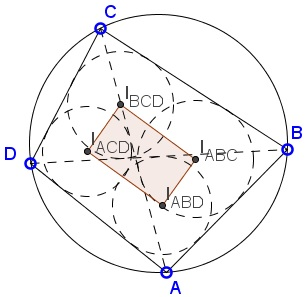# Incenters in Cyclic Quadrilateral: What is this about? A Mathematical Droodle

ExplanationEvery (convex) quadrilateral ABCD defines four triangles: BCD, ACD, ABD, and ABC. The applet is an attempt to convey an assertion often referred to as the Japanese theorem:

The incenters of the four triangles form a rectangle.### Proof

From ΔBCD, ∠BIBCDC = 90° + ∠BDC/2.

Similarly, from ΔABC, ∠BIABCC = 90° + ∠BAC/2.

However, since we assumed that the quadrilateral ABCD is cyclic, ∠BDC = ∠BAC. Therefore, also ∠BIBCDC = ∠BIABCC. The quadrilateral BIABCIBCDC is therefore cyclic, so that

 (1) ∠BCIBCD + ∠BIABCIBCD = 180°.

Similarly the quadrilateral AIABDIABCB is cyclic, so that

 (2) ∠BAIABD + ∠BIABCIABD = 180°.

(1) and (2) imply that

∠BIABCIBCD + ∠BIABCIABD = 360° - ∠BCD/2 - ∠BAD/2 = 270°.

Which gives

 (3) ∠IABDIABCIBCD = 90°.

Other angles are treated similarly. Q.E.D.

In addition, let P, Q, R, and S be the midpoints of the arcs AB, CD, BC, and AD, respectively.It's almost immediate to prove that PQ⊥RS. This is also true that PQ and RS are parallel to the sides of the rectangle of the incenters and pass through their midpoints.

This is #3.5 from Fukagawa and Pedoe's collection. It was written on a 1880 tablet in the Yamagata prefecture. Maruyama Ryoukan posted a tablet in 1800 at the Sannosha shrine in Tsuruoka city of Yamagata prefecture with the observation that [Fukagawa and Rothman, p. 192]

rABC + rACD = rABD + rBCD.

(In itself, this is a particular case of what is known as the Old Japanese Theorem.)

This implies that the lines through the incenters parallel to the diagonals form a rhombus.You may observe that the arc bisectors of the arcs subtended by the sides of the quadrilateral serve as the diagonals of the rhombus.

### References

1. H. Fukagawa, D. Pedoe, Japanese Temple Geometry Problems, The Charles Babbage Research Center, Winnipeg, 1989

Write to:

Charles Babbage Research Center
P.O. Box 272, St. Norbert Postal Station
Winnipeg, MB